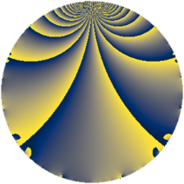# Properties

 Label 1734.2.aLevel $1734$ Weight $2$ Character orbit 1734.a Rep. character $\chi_{1734}(1,\cdot)$ Character field $\Q$ Dimension $45$ Newform subspaces $23$ Sturm bound $612$ Trace bound $7$

# Related objects

## Defining parameters

 Level: $$N$$ $$=$$ $$1734 = 2 \cdot 3 \cdot 17^{2}$$ Weight: $$k$$ $$=$$ $$2$$ Character orbit: $$[\chi]$$ $$=$$ 1734.a (trivial) Character field: $$\Q$$ Newform subspaces: $$23$$ Sturm bound: $$612$$ Trace bound: $$7$$ Distinguishing $$T_p$$: $$5$$, $$7$$

## Dimensions

The following table gives the dimensions of various subspaces of $$M_{2}(\Gamma_0(1734))$$.

Total New Old
Modular forms 342 45 297
Cusp forms 271 45 226
Eisenstein series 71 0 71

The following table gives the dimensions of the cuspidal new subspaces with specified eigenvalues for the Atkin-Lehner operators and the Fricke involution.

$$2$$$$3$$$$17$$FrickeDim.
$$+$$$$+$$$$+$$$$+$$$$3$$
$$+$$$$+$$$$-$$$$-$$$$8$$
$$+$$$$-$$$$+$$$$-$$$$6$$
$$+$$$$-$$$$-$$$$+$$$$5$$
$$-$$$$+$$$$+$$$$-$$$$8$$
$$-$$$$+$$$$-$$$$+$$$$4$$
$$-$$$$-$$$$+$$$$+$$$$2$$
$$-$$$$-$$$$-$$$$-$$$$9$$
Plus space$$+$$$$14$$
Minus space$$-$$$$31$$

## Trace form

 $$45q + q^{2} - q^{3} + 45q^{4} + 6q^{5} - q^{6} + q^{8} + 45q^{9} + O(q^{10})$$ $$45q + q^{2} - q^{3} + 45q^{4} + 6q^{5} - q^{6} + q^{8} + 45q^{9} - 2q^{10} + 4q^{11} - q^{12} + 10q^{13} + 2q^{15} + 45q^{16} + q^{18} + 6q^{20} - 4q^{21} + 4q^{22} - q^{24} + 47q^{25} - 2q^{26} - q^{27} + 14q^{29} + 6q^{30} + 8q^{31} + q^{32} + 8q^{33} + 45q^{36} - 2q^{37} + 4q^{38} - 6q^{39} - 2q^{40} - 6q^{41} + 4q^{42} + 8q^{43} + 4q^{44} + 6q^{45} - 8q^{47} - q^{48} + 69q^{49} + 15q^{50} + 10q^{52} - 2q^{53} - q^{54} + 4q^{57} + 6q^{58} + 4q^{59} + 2q^{60} + 6q^{61} - 24q^{62} + 45q^{64} - 28q^{65} + 4q^{66} + 40q^{67} + 16q^{69} + 16q^{70} + q^{72} - 14q^{73} + 6q^{74} + 17q^{75} + 8q^{77} + 10q^{78} + 8q^{79} + 6q^{80} + 45q^{81} - 14q^{82} + 4q^{83} - 4q^{84} - 12q^{86} + 10q^{87} + 4q^{88} + 42q^{89} - 2q^{90} - 16q^{91} - 4q^{93} + 24q^{94} + 24q^{95} - q^{96} - 6q^{97} + 9q^{98} + 4q^{99} + O(q^{100})$$

## Decomposition of $$S_{2}^{\mathrm{new}}(\Gamma_0(1734))$$ into newform subspaces

Label Dim. $$A$$ Field CM Traces A-L signs $q$-expansion
$$a_2$$ $$a_3$$ $$a_5$$ $$a_7$$ 2 3 17
1734.2.a.a $$1$$ $$13.846$$ $$\Q$$ None $$-1$$ $$-1$$ $$-1$$ $$4$$ $$+$$ $$+$$ $$-$$ $$q-q^{2}-q^{3}+q^{4}-q^{5}+q^{6}+4q^{7}+\cdots$$
1734.2.a.b $$1$$ $$13.846$$ $$\Q$$ None $$-1$$ $$-1$$ $$0$$ $$-2$$ $$+$$ $$+$$ $$+$$ $$q-q^{2}-q^{3}+q^{4}+q^{6}-2q^{7}-q^{8}+\cdots$$
1734.2.a.c $$1$$ $$13.846$$ $$\Q$$ None $$-1$$ $$-1$$ $$0$$ $$1$$ $$+$$ $$+$$ $$+$$ $$q-q^{2}-q^{3}+q^{4}+q^{6}+q^{7}-q^{8}+\cdots$$
1734.2.a.d $$1$$ $$13.846$$ $$\Q$$ None $$-1$$ $$-1$$ $$2$$ $$2$$ $$+$$ $$+$$ $$+$$ $$q-q^{2}-q^{3}+q^{4}+2q^{5}+q^{6}+2q^{7}+\cdots$$
1734.2.a.e $$1$$ $$13.846$$ $$\Q$$ None $$-1$$ $$1$$ $$-2$$ $$-2$$ $$+$$ $$-$$ $$+$$ $$q-q^{2}+q^{3}+q^{4}-2q^{5}-q^{6}-2q^{7}+\cdots$$
1734.2.a.f $$1$$ $$13.846$$ $$\Q$$ None $$-1$$ $$1$$ $$0$$ $$-1$$ $$+$$ $$-$$ $$-$$ $$q-q^{2}+q^{3}+q^{4}-q^{6}-q^{7}-q^{8}+\cdots$$
1734.2.a.g $$1$$ $$13.846$$ $$\Q$$ None $$-1$$ $$1$$ $$1$$ $$-4$$ $$+$$ $$-$$ $$+$$ $$q-q^{2}+q^{3}+q^{4}+q^{5}-q^{6}-4q^{7}+\cdots$$
1734.2.a.h $$1$$ $$13.846$$ $$\Q$$ None $$-1$$ $$1$$ $$4$$ $$2$$ $$+$$ $$-$$ $$+$$ $$q-q^{2}+q^{3}+q^{4}+4q^{5}-q^{6}+2q^{7}+\cdots$$
1734.2.a.i $$1$$ $$13.846$$ $$\Q$$ None $$1$$ $$-1$$ $$-4$$ $$-3$$ $$-$$ $$+$$ $$+$$ $$q+q^{2}-q^{3}+q^{4}-4q^{5}-q^{6}-3q^{7}+\cdots$$
1734.2.a.j $$1$$ $$13.846$$ $$\Q$$ None $$1$$ $$-1$$ $$2$$ $$0$$ $$-$$ $$+$$ $$+$$ $$q+q^{2}-q^{3}+q^{4}+2q^{5}-q^{6}+q^{8}+\cdots$$
1734.2.a.k $$1$$ $$13.846$$ $$\Q$$ None $$1$$ $$-1$$ $$3$$ $$4$$ $$-$$ $$+$$ $$+$$ $$q+q^{2}-q^{3}+q^{4}+3q^{5}-q^{6}+4q^{7}+\cdots$$
1734.2.a.l $$1$$ $$13.846$$ $$\Q$$ None $$1$$ $$1$$ $$-3$$ $$-4$$ $$-$$ $$-$$ $$-$$ $$q+q^{2}+q^{3}+q^{4}-3q^{5}+q^{6}-4q^{7}+\cdots$$
1734.2.a.m $$1$$ $$13.846$$ $$\Q$$ None $$1$$ $$1$$ $$4$$ $$3$$ $$-$$ $$-$$ $$-$$ $$q+q^{2}+q^{3}+q^{4}+4q^{5}+q^{6}+3q^{7}+\cdots$$
1734.2.a.n $$2$$ $$13.846$$ $$\Q(\sqrt{2})$$ None $$2$$ $$-2$$ $$4$$ $$4$$ $$-$$ $$+$$ $$+$$ $$q+q^{2}-q^{3}+q^{4}+(2+\beta )q^{5}-q^{6}+\cdots$$
1734.2.a.o $$2$$ $$13.846$$ $$\Q(\sqrt{2})$$ None $$2$$ $$2$$ $$-4$$ $$-4$$ $$-$$ $$-$$ $$+$$ $$q+q^{2}+q^{3}+q^{4}+(-2+\beta )q^{5}+q^{6}+\cdots$$
1734.2.a.p $$3$$ $$13.846$$ $$\Q(\zeta_{18})^+$$ None $$-3$$ $$-3$$ $$-6$$ $$-3$$ $$+$$ $$+$$ $$-$$ $$q-q^{2}-q^{3}+q^{4}+(-2+\beta _{1})q^{5}+q^{6}+\cdots$$
1734.2.a.q $$3$$ $$13.846$$ $$\Q(\zeta_{18})^+$$ None $$-3$$ $$3$$ $$6$$ $$3$$ $$+$$ $$-$$ $$+$$ $$q-q^{2}+q^{3}+q^{4}+(2-\beta _{1})q^{5}-q^{6}+\cdots$$
1734.2.a.r $$3$$ $$13.846$$ $$\Q(\zeta_{18})^+$$ None $$3$$ $$-3$$ $$-6$$ $$3$$ $$-$$ $$+$$ $$+$$ $$q+q^{2}-q^{3}+q^{4}+(-2+\beta _{1})q^{5}-q^{6}+\cdots$$
1734.2.a.s $$3$$ $$13.846$$ $$\Q(\zeta_{18})^+$$ None $$3$$ $$3$$ $$6$$ $$-3$$ $$-$$ $$-$$ $$-$$ $$q+q^{2}+q^{3}+q^{4}+(2-\beta _{1})q^{5}+q^{6}+\cdots$$
1734.2.a.t $$4$$ $$13.846$$ $$\Q(\zeta_{16})^+$$ None $$-4$$ $$-4$$ $$8$$ $$0$$ $$+$$ $$+$$ $$-$$ $$q-q^{2}-q^{3}+q^{4}+(2+\beta _{2}+\beta _{3})q^{5}+\cdots$$
1734.2.a.u $$4$$ $$13.846$$ $$\Q(\zeta_{16})^+$$ None $$-4$$ $$4$$ $$-8$$ $$0$$ $$+$$ $$-$$ $$-$$ $$q-q^{2}+q^{3}+q^{4}+(-2-\beta _{2}+\beta _{3})q^{5}+\cdots$$
1734.2.a.v $$4$$ $$13.846$$ $$\Q(\zeta_{16})^+$$ None $$4$$ $$-4$$ $$0$$ $$-8$$ $$-$$ $$+$$ $$-$$ $$q+q^{2}-q^{3}+q^{4}+(-\beta _{2}+\beta _{3})q^{5}+\cdots$$
1734.2.a.w $$4$$ $$13.846$$ $$\Q(\zeta_{16})^+$$ None $$4$$ $$4$$ $$0$$ $$8$$ $$-$$ $$-$$ $$-$$ $$q+q^{2}+q^{3}+q^{4}+(\beta _{2}+\beta _{3})q^{5}+q^{6}+\cdots$$

## Decomposition of $$S_{2}^{\mathrm{old}}(\Gamma_0(1734))$$ into lower level spaces

$$S_{2}^{\mathrm{old}}(\Gamma_0(1734)) \cong$$ $$S_{2}^{\mathrm{new}}(\Gamma_0(17))$$$$^{\oplus 8}$$$$\oplus$$$$S_{2}^{\mathrm{new}}(\Gamma_0(34))$$$$^{\oplus 4}$$$$\oplus$$$$S_{2}^{\mathrm{new}}(\Gamma_0(51))$$$$^{\oplus 4}$$$$\oplus$$$$S_{2}^{\mathrm{new}}(\Gamma_0(102))$$$$^{\oplus 2}$$$$\oplus$$$$S_{2}^{\mathrm{new}}(\Gamma_0(289))$$$$^{\oplus 4}$$$$\oplus$$$$S_{2}^{\mathrm{new}}(\Gamma_0(578))$$$$^{\oplus 2}$$$$\oplus$$$$S_{2}^{\mathrm{new}}(\Gamma_0(867))$$$$^{\oplus 2}$$